# 1. Let x, a € R. Prove that if a <a, then -a < x <a.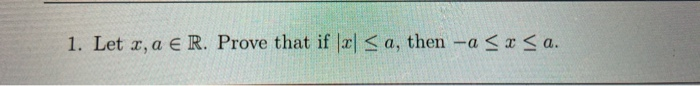1. Let x, a € R. Prove that if a <a, then -a < x <a.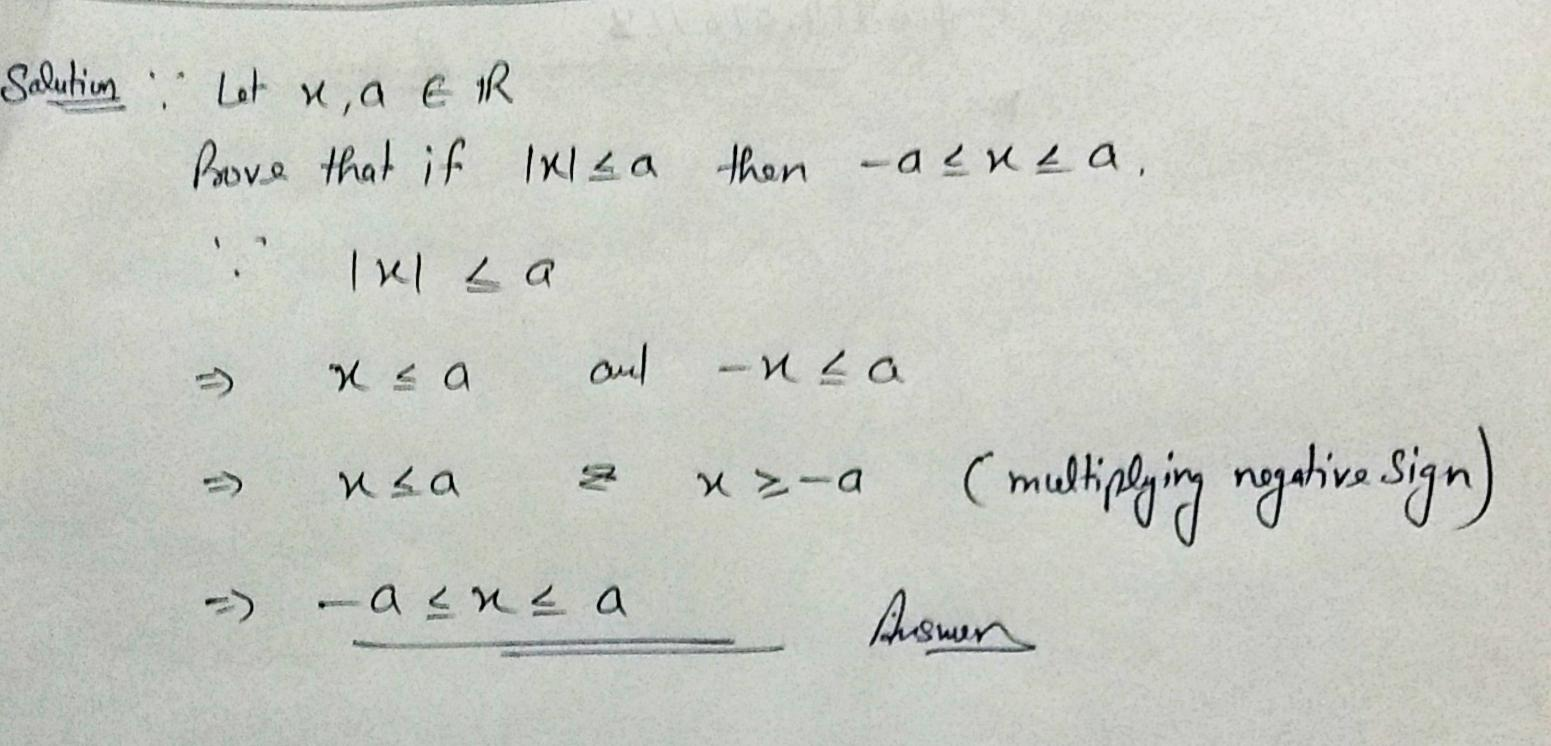##### Add Answer of: 1. Let x, a € R. Prove that if a <a, then -a < x <a.
Similar Homework Help Questions
• ### Let f and g be differentiable on R such that f(1) = g(1), and f'(x) <...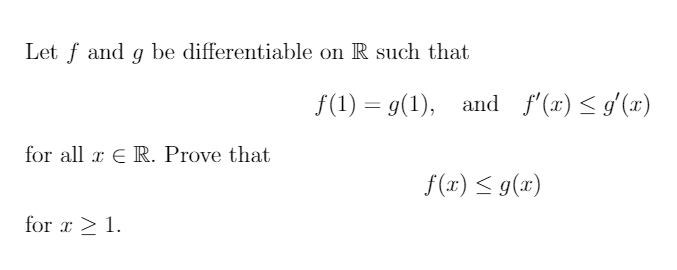Let f and g be differentiable on R such that f(1) = g(1), and f'(x) < '() for all r ER. Prove that f(x) = g(2) for 3 >1.

• ### an (4) Let F be ordered field. Prove that the statement Vo: ZX\x6F*XWye FtX &<y)->(<y') is...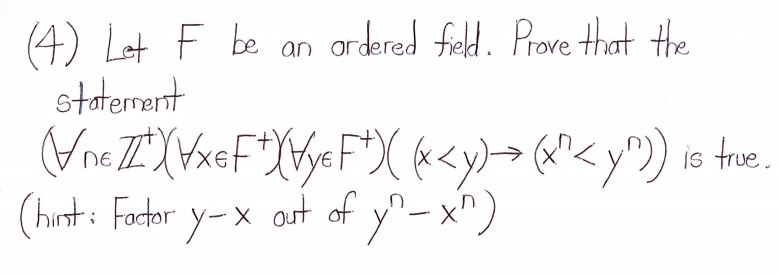an (4) Let F be ordered field. Prove that the statement Vo: ZX\x6F*XWye FtX &<y)->(<y') is true (hist: Factor Y-X out of yº-x")

• ### x" dx TC 15. (a) So 1 + x x for 0 < a < 1....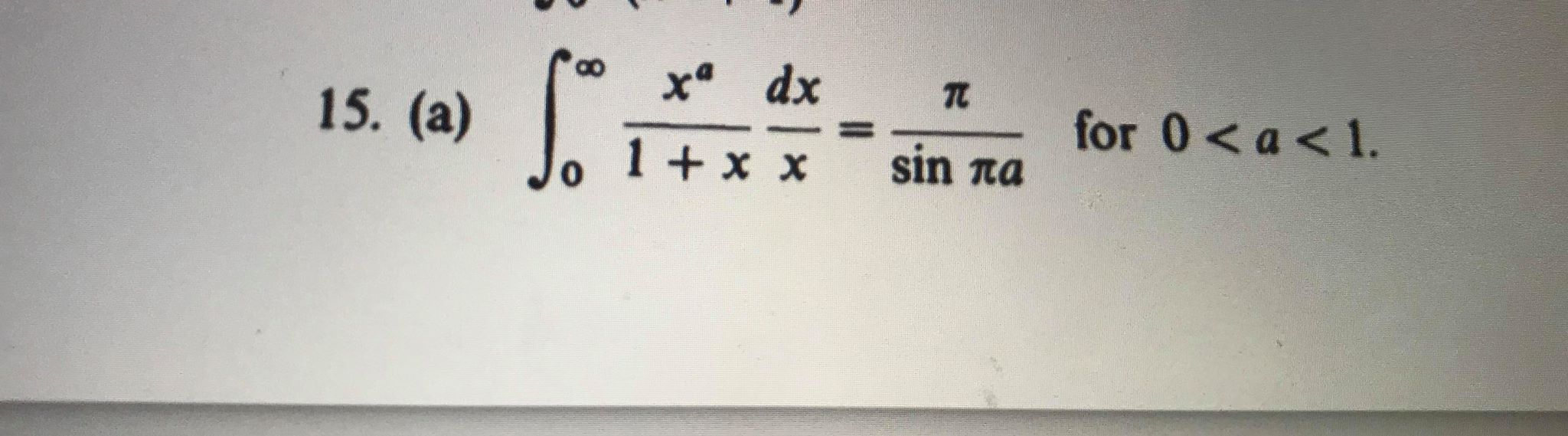x" dx TC 15. (a) So 1 + x x for 0 < a < 1. sin πα

• ### It is known that f :(0,2) + R is a differentiable function such that \f'(x) <...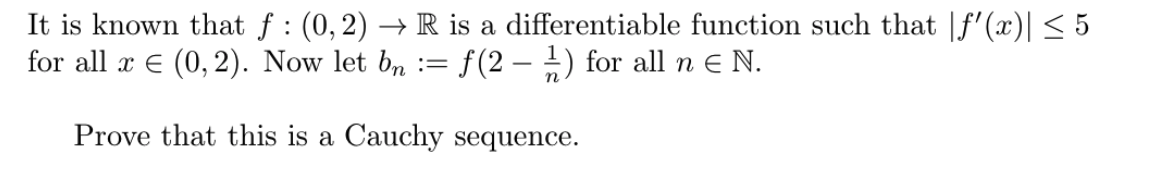It is known that f :(0,2) + R is a differentiable function such that \f'(x) < 5 for all x € (0,2). Now let bn := f(2 – †) for all n € N. Prove that this is a Cauchy sequence.

• ### The following pdf is given for 0<x<2 f;(r) =. - 0. N. Let Y = 4...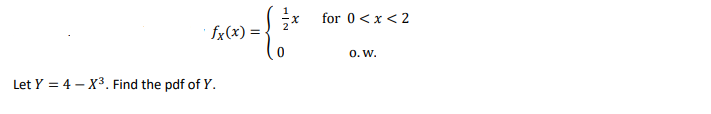The following pdf is given for 0<x<2 f;(r) =. - 0. N. Let Y = 4 - X3. Find the pdf of Y.

• ### 3. (15 pts) Let D be an infinite set with cardinal d. Let A = {X...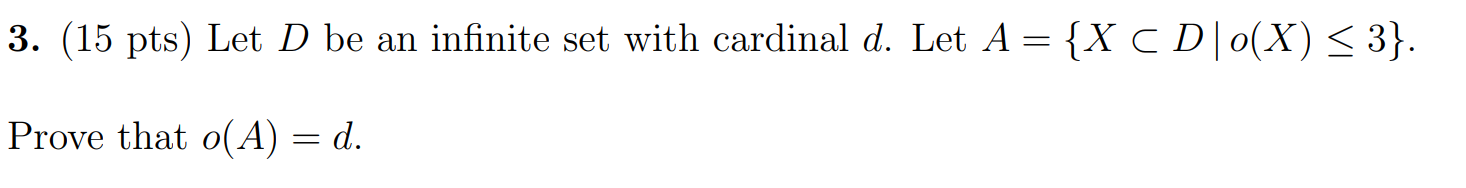3. (15 pts) Let D be an infinite set with cardinal d. Let A = {X C D | 0(X) <3}. Prove that o(A) = d.

• ### 2. Let f(x) = 8 + 3x4 -1 5x<1, f(x+2) = f(x). Which best describes the...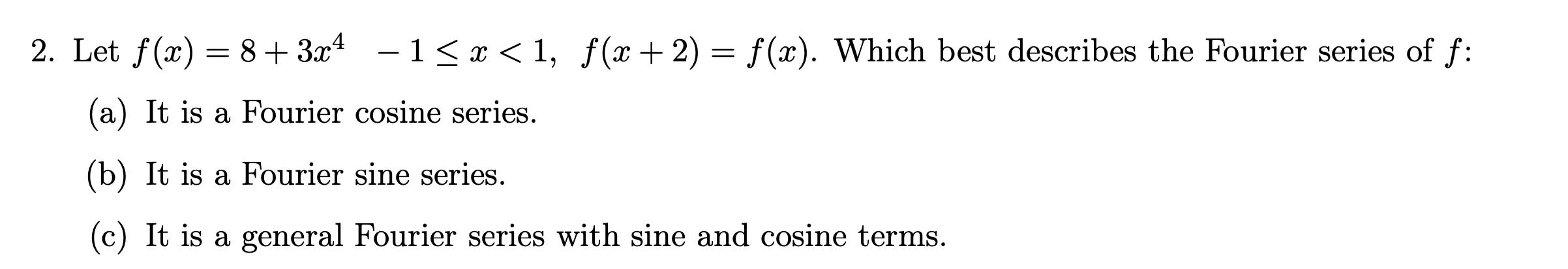2. Let f(x) = 8 + 3x4 -1 5x<1, f(x+2) = f(x). Which best describes the Fourier series of f: (a) It is a Fourier cosine series. (b) It is a Fourier sine series. (c) It is a general Fourier series with sine and cosine terms.

• ### 1 xe Let f(x)={? x 8. Prove that f(x) continuous only at +1. Let f(x)= \$3.x...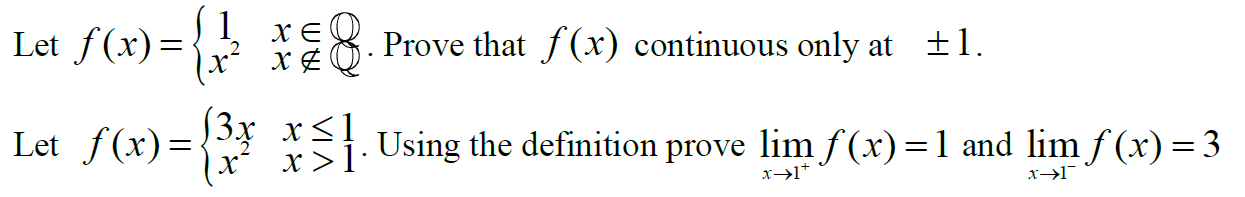1 xe Let f(x)={? x 8. Prove that f(x) continuous only at +1. Let f(x)= \$3.x xs! x >1 Using the definition prove lim f(x)=1 and lim f (x) = 3 x>17 11°

• ### 9. The distribution function of a random variable X is given by 0, for r<-1, F(x)...9. The distribution function of a random variable X is given by 0, for r<-1, F(x) = { 271 -1<x<1, 1, 2 > 1. Find (a) P(Z < X < }); (b) P(1<x< 2).

• ### Prove X, Y, Z, JER. XKY Prove Z <# X AND Y<j, if and only if...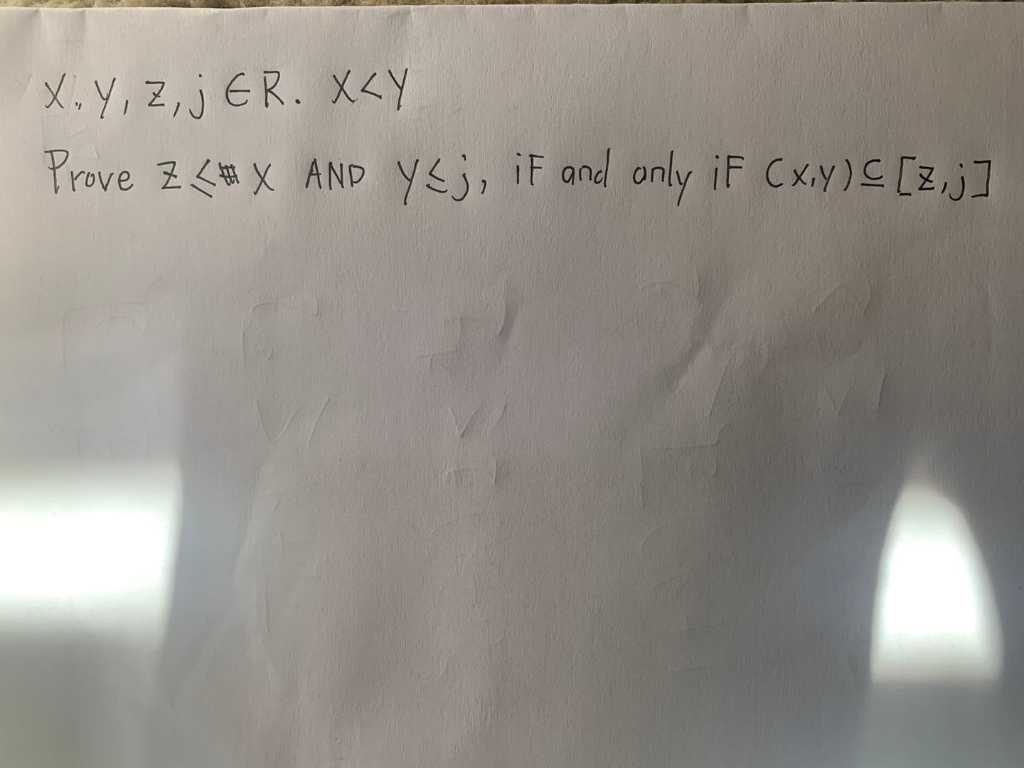Prove X, Y, Z, JER. XKY Prove Z <# X AND Y<j, if and only if (x,y) [z,j]

Free Homework App